# Translations of Encyclopedia about Mathematics

## Differential Calculus, Derivative

The foundations of differential calculus were laid by German mathematician and physicist Gorttfried W. Leibnitz (1646-1716) and English physicist and mathematician Isaac Newton (1643-1727). It was primarily Newton’s discovered binomial theorem for discrete functions which enabled the creation of differential calculus. Leibnitz studied problems concerning tangents, setting the presently used form of expressions for derivative quotients and integrals.

Newton invented fluxion calculus for deriving the gravitational law and which he created based on laws concerning the movement of planets as laid out by Johann Kepler (1571-1630). Both these fields were developed further and influenced each other, although the concept of limits was not yet developed. It was only until the 19th century when the concept of limits was introduced by mathematicians Louis A. Cauchy (1789-1857), Karl Weierstrass (1815-1897), Bernhard Riemann (1826-1866) and Richard Dedekind (1831-1910) and when the infinitesimal method of thinking developed to clarify work with it.

With the help of analytical geometry, derivative geometry was advanced, a different field of derivative calculus which influenced the entire field of physics and technology. Without the theory of real and complex functions and differential calculus, they would not be conceivable today. Derivatives also affected the foundations of mathematics and the need arose to mathematically and more clearly describe continual processes.

If some curve exists in a square coordinate system, the characteristics of the function y = f(x), where x is a real variable, can be examined using real numbers. For example, the speed of a vehicle is larger the steeper is the curve in a coordinate diagram of distance and time.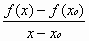From this, it follows that, in the interval, there is a linear ratio of growth, referred to as the derivative of the function f: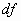. The statement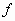´ ==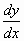oris considered a derivative quotient or the first derivative of function (x) and expresses the behaviour of the curve at point Px. This function can be further differentiated to give a second derivative function.

At the point x = x0, the function is considered as differentiable only if there exists a left and right limit to the differential quotient and if they are equal. If some function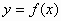is differentiable at the point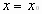, it is also continuous at that point.

By differentiation, the extremes of a function, also referred to as its maximum and minimum, can also be determined. This while the second or third non-zero derivative can show whether it is truly a maximum or minimum.

The maximum for xmax is when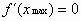and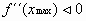The minimum for xmin is whenandThese conditions guarantee the existence of a minimum or maximum.

There also exist certain conditions which must be fulfilled for the inverse point of a function.

The inverse point foris whenand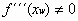A function or its graph are concave (convex) in an interval (x1; x2) if the graph is curved in the direction to the right (left) and if its second derivative is negative (positive).

The conditions for a tangent in an inverse point are:whereIf at some point a function does not have a functional value, thenand the curve of the graph can pass through the axis x at that point.

Translating German French Translations French German Translating Polish German Translations English French Translating PolishEnter your search terms Submit search form